Home

# On the propagation of gravity currents over and through a submerged array of circular cylinders

## Abstract

The propagation of full-depth lock-exchange bottom gravity currents past a submerged array of circular cylinders is investigated using laboratory experiments and large eddy simulations. Firstly, to investigate the front velocity of gravity currents across the whole range of array density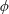$\unicode[STIX]{x1D719}$ (i.e. the volume fraction of solids), the array is densified from a flat bed (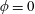$\unicode[STIX]{x1D719}=0$ ) towards a solid slab (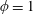$\unicode[STIX]{x1D719}=1$ ) under a particular submergence ratio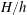$H/h$ , where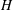$H$ is the flow depth and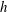$h$ is the array height. The time-averaged front velocity in the slumping phase of the gravity current is found to first decrease and then increase with increasing$\unicode[STIX]{x1D719}$ . Next, a new geometrical framework consisting of a streamwise array density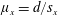$\unicode[STIX]{x1D707}_{x}=d/s_{x}$ and a spanwise array density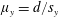$\unicode[STIX]{x1D707}_{y}=d/s_{y}$ is proposed to account for organized but non-equidistant arrays (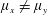$\unicode[STIX]{x1D707}_{x}\neq \unicode[STIX]{x1D707}_{y}$ ), where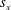$s_{x}$ and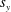$s_{y}$ are the streamwise and spanwise cylinder spacings, respectively, and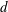$d$ is the cylinder diameter. It is argued that this two-dimensional parameter space can provide a more quantitative and unambiguous description of the current–array interaction compared with the array density given by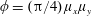$\unicode[STIX]{x1D719}=(\unicode[STIX]{x03C0}/4)\unicode[STIX]{x1D707}_{x}\unicode[STIX]{x1D707}_{y}$ . Both in-line and staggered arrays are investigated. Four dynamically different flow regimes are identified: (i) through-flow propagating in the array interior subject to individual cylinder wakes (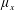$\unicode[STIX]{x1D707}_{x}$ : small for in-line array and arbitrary for staggered array;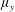$\unicode[STIX]{x1D707}_{y}$ : small); (ii) over-flow propagating on the top of the array subject to vertical convective instability ($\unicode[STIX]{x1D707}_{x}$ : large;$\unicode[STIX]{x1D707}_{y}$ : large); (iii) plunging-flow climbing sparse close-to-impermeable rows of cylinders with minor streamwise intrusion ($\unicode[STIX]{x1D707}_{x}$ : small;$\unicode[STIX]{x1D707}_{y}$ : large); and (iv) skimming-flow channelized by an in-line array into several subcurrents with strong wake sheltering ($\unicode[STIX]{x1D707}_{x}$ : large;$\unicode[STIX]{x1D707}_{y}$ : small). The most remarkable difference between in-line and staggered arrays is the non-existence of skimming-flow in the latter due to the flow interruption by the offset rows. Our analysis reveals that as$\unicode[STIX]{x1D719}$ increases, the change of flow regime from through-flow towards over- or skimming-flow is responsible for increasing the gravity current front velocity.

## References

Hide All
MathJax
MathJax is a JavaScript display engine for mathematics. For more information see http://www.mathjax.org.

# On the propagation of gravity currents over and through a submerged array of circular cylinders

## Metrics

### Full text viewsFull text views reflects the number of PDF downloads, PDFs sent to Google Drive, Dropbox and Kindle and HTML full text views.

Total number of HTML views: 0
Total number of PDF views: 0 *Loading metrics...

### Abstract viewsAbstract views reflect the number of visits to the article landing page.

Total abstract views: 0 *Loading metrics...

* Views captured on Cambridge Core between <date>. This data will be updated every 24 hours.

Usage data cannot currently be displayed.